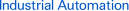Page topresidual voltage

Definition

The appropriate product category
The residual voltage is the voltage that remains in an output transistor while it is ON.
For example, consider an NPN output with a power supply voltage of 24 V and a residual voltage of 2 V. While the output transistor is ON, there will be 2 V between the output line (the black wire) and the 0-V line (the blue wire).
A relay, timer, or other load is connected between the power supply line (the brown wire) and the output line (the black wire).
A voltage of 22 V is applied to the load at this time.
(Voltage across the load of 22 V = Power supply voltage of 24 V - Residual voltage of 2 V)